Latest Banking jobs   »   Quantitative Aptitude Quiz For IBPS RRB...

# Quantitative Aptitude Quiz For IBPS RRB PO, Clerk Prelims 2021- 11th May

Directions (1–5): Simplify the following questions and find the value of (?)

Q1. 24 × 18 + 560 ÷ 14 = 600 – ?
(a) 132
(b) 128
(c) 140
(d) 146
(e) 152

Q2. 2685 – 1460 + 737 = 1793 + (?)²
(a) 12
(b) 14
(c) 13
(d) 11
(e) None of these

Q3. 780 ÷ 12 × 14 + 220 = ?
(a) 1130
(b) 1140
(c) 1155
(d) 1170
(e) 1148

Q4. 840 ÷ 14 + 28 × 14 + (?)² = 533
(a) 10
(b) 8
(c)11
(d) 9
(e) None of these

Q5. 3120 ÷ 24 + 1650 + ? = 2180
(a) 420
(b) 430
(c) 440
(d) 450
(e) 400

Directions (6-10): In each of the following questions two equations (I) and (II) are given, you have to solve both the equation and give answer.
(a) if x < y
(b) if x ≤ y
(c) if x > y
(d) if x ≥ y
(e) if x = y or no relationship can be obtained between x and y.

Q6. (I) x² – 14x + 48 = 0
(II) y² – 18y + 80 = 0

Q7. (I) x³ + 328 = 2525
(II) y³ + 349 = 1680

Q8. (I) x² – 19x + 88 = 0
(II) y² – 21y + 108 = 0

Q9. (I) x³ = 1728
(II) y² = 144

Q10. (I) 2x² + 25x + 75 = 0
(II) 3y² + 26y + 56 = 0

Directions (11-15): The given pie chart shows the distribution (in degree) of total monthly income of a person into 6 different categories. Study the graph carefully and answer the following questions.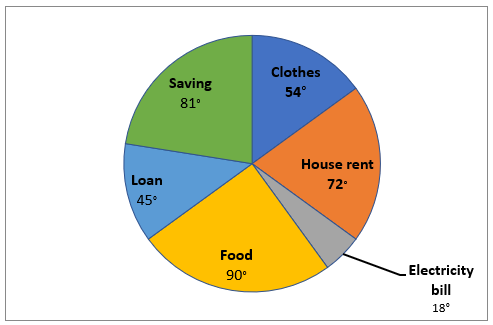Note – Consider all categories as monthly expenditure of the person except savings.

Q11. If monthly salary is Rs 18000, find monthly expenditure on food?
(a) Rs 7200
(b) Rs 5400
(c) Rs 4500
(d) Rs 6300
(e) Rs 6800

Q12. If difference between monthly expenditure on House rent and Clothes is Rs 2800, then find monthly income of person.
(a) Rs 48000
(b) Rs 56000
(c) Rs 54000
(d) Rs 42000
(e) Rs 62000

Q13. Find the average of monthly expenditure on Food, Clothes and House rent, if monthly expenditure on Loan is Rs 3600.
(a) Rs 5760
(b) Rs 4670
(c) Rs 6370
(d) Rs 5670
(e) Rs 5360

Q14. What is the total monthly expenditure if monthly income of the person is Rs 84000?
(a) Rs 65000
(b) Rs 64900
(c) Rs 65900
(d) Rs 65100
(e) Rs 64100

Q15. Monthly expenditure on Clothes is what percent of monthly expenditure on House rent?
(a) 90%
(b) 75%
(c) 50%
(d) 66.67%
(e) 133.33%

Practice More Questions of Quantitative Aptitude for Competitive Exams:

###### Study Plan for IBPS RRB PO/Clerk Prelims 2021

Solutions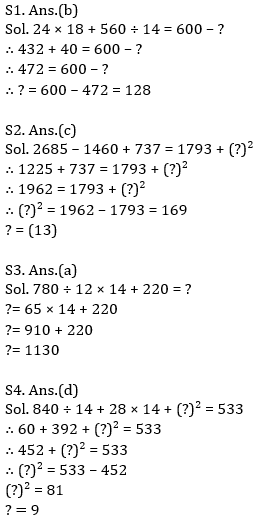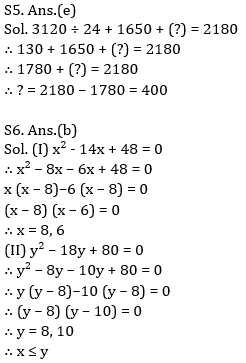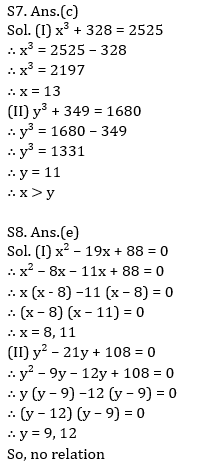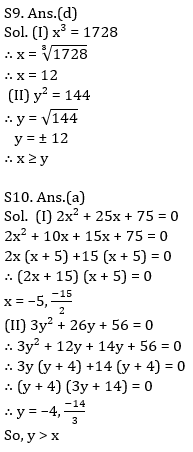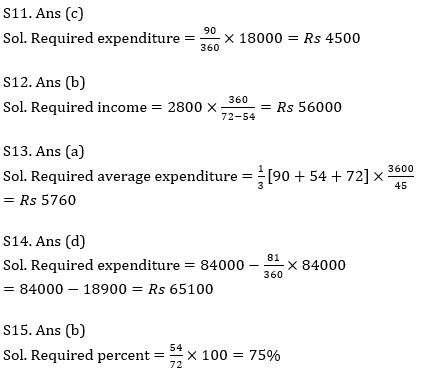#### Congratulations!Incorrect details? Fill the form again here

•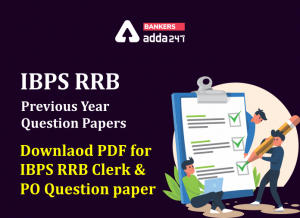IBPS RRB Previous Year Question Paper PD...
•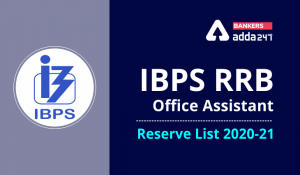IBPS RRB Clerk 2nd Reserve List 2022 Out...
•Quantitative Aptitude Quiz For IBPS RRB ...
•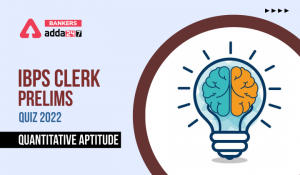Quantitative Aptitude Quiz For IBPS Cler...
•Reasoning Ability Quiz For IBPS RRB PO P...
•Quantitative Aptitude Quiz For IBPS RRB ...